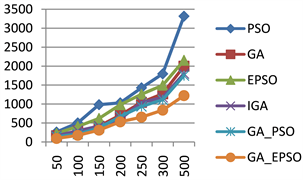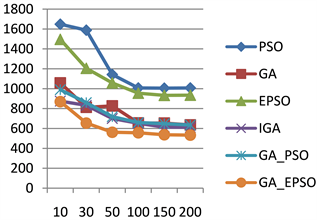﻿ 基于云计算任务调度的遗传粒子群优化算法

# 基于云计算任务调度的遗传粒子群优化算法Genetic and Particle Swarm Optimization Algorithm Based on Cloud Task Scheduling

Abstract: The task scheduling algorithm of cloud platform is a hot topic in the field of cloud computing. How to achieve faster convergence speed while not meeting the local optimal solution has always been one of the goals pursued by researchers. To this end, this paper proposes an enhanced genetic and particle swarm optimization algorithm (GA_EPSO) that introduces an enhanced particle swarm optimization algorithm (EPSO) with improved random factors and inertia weights into mutation operations in genetic algorithm (GA). Reconstructing the mutation operator by the current optimal solution and the global optimal solution in enhanced particle swarm optimization algorithm, the enhanced genetic and particle swarm optimization algorithm has a faster convergence speed without falling into the local optimal solution. Simulation experiments show that under the same conditions, compared with genetic algorithm (GA), improved genetic algorithm (IGA), particle swarm optimization (PSO), enhanced particle swarm optimization (EPSO) and genetic particle swarm optimization (GA_PSO), the algorithm not only accelerates the convergence speed, but also has a significant improvement in task scheduling efficiency.

1. 引言

2. 相关工作

3. 云计算任务调度问题分析

4. 基于增强型遗传粒子群混合算法(GA_EPSO)的任务调度

4.1. 算法建模

1) 任务的长度在一定范围内随机选取；

2) 所有云计算资源被映射成虚拟机，且虚拟机的性能在一定范围内随机选取；

3) 提交的任务数量远远大于虚拟机数量。

4.2. 遗传编码

4.3. 算法设计思想

4.4. 适应度函数

$\text{fitness}=\mathrm{max}\left({\text{time}}_{i}\right),i\in \left\{0,1,2,\cdots ,n-1\right\}$ (1)

4.5. 遗传操作

${p}_{i}=1/\left(f\left(i\right)\ast \theta \right)i\in \left(1,2,3,\cdots ,n\right)$ (2)

$\theta ={\sum }_{i=1}^{n}f\left(i\right),i\in \left(0,1,2,\cdots ,n\right)$ (3)

${v}_{id}\left(t+1\right)=\omega {v}_{id}\left(t\right)+{c}_{1}{r}_{1}\left(t\right)\left({p}_{id}\left(t\right)-{x}_{id}\left(t\right)\right)+{c}_{2}{r}_{2}\left(t\right)\left({p}_{gd}\left(t\right)-{x}_{id}\left(t\right)\right)$ (4)

${x}_{id}\left(t+1\right)={x}_{id}\left(t\right)+{v}_{id}\left(t+1\right)$ (5)

$\omega \left(t\right)=\left({\omega }_{\mathrm{max}}-{\omega }_{\mathrm{min}}\right){p}_{s}\left(t\right)+{\omega }_{\mathrm{min}}0\le {\omega }_{\mathrm{min}}\le {\omega }_{\mathrm{max}}$ (6)

${p}_{s}\left(t\right)={\sum }_{i=1}^{n}S\left(i,t\right)/n$ (7)

$S\left(i,t\right)=\left\{\begin{array}{c}1,\text{\hspace{0.17em}}\text{fitness}\left({\text{pbest}}_{i}^{t}\right)<\text{fitness}\left({\text{pbest}}_{i}^{t-1}\right)\\ 且\text{fitness}\left({\text{pbest}}_{i}^{t}\right)<{\text{globalbest}}^{t};\\ 0.5,\text{\hspace{0.17em}}\text{fitness}\left({\text{pbest}}_{i}^{t}\right)<\text{fitness}\left({\text{pbest}}_{i}^{t-1}\right)\\ 且\text{fitness}\left({\text{pbest}}_{i}^{t}\right)\ge {\text{globalbest}}^{t};\\ 0,\text{\hspace{0.17em}}\text{fitness}\left({\text{pbest}}_{i}^{t}\right)=\text{fitness}\left({\text{pbest}}_{i}^{t-1}\right)\end{array}$ (8)

5. 仿真试验与分析

5.1. 实验环境及参数设置

5.2. 实验结果分析Table 1. Algorithm parameter settingFigure 1. Comparison of timeFigure 2. Comparison of convergence

6. 结束语

NOTES

*通讯作者。

 Ravi Kumar, P., Herbert Raj, P. and Jelciana, P. (2017) Exploring Security Issues and Solutions in Cloud Computing Services—A Survey. Cybernetics and Information Technologies, 17, 55-59.

 Vose, M.D. (1999) The Simple Ge-netic Algorithm. MIT Press, Cambridge, 31-57.

 葛继科, 邱玉辉, 吴春明, 等. 遗传算法研究综述[J]. 计算机应用研究, 2008(10): 2911-2916.

 马永杰, 云文霞. 遗传算法研究进展[J]. 计算机应用研究, 2012, 29(4): 1201-1206, 1210.

 戴朝华. 搜寻者优化算法及其应用研究[D]: [博士学位论文]. 成都: 西南交通大学, 2010.

 匡芳君. 智能混合优化算法及其应用研究[D]: [博士学位论文]. 南京: 南京理工大学, 2014.

 张雨, 李芳, 周涛. 云计算环境下基于遗传蚁群算法的任务调度研究[J]. 计算机工程与应用, 2014, 50(6): 51-55.

 徐洁, 朱健琛, 鲁珂. 基于双适应度遗传退火的云任务调度算法[J]. 电子科技大学学报, 2013, 42(6): 900-904.

 王波, 张晓磊. 基于粒子群遗传算法的云计算任务调度研究[J]. 计算机工程与应用, 2015, 51(6): 84-88.

 陈璐璐, 邱建林, 陈燕云, 等. 改进的遗传粒子群混合优化算法[J]. 计算机工程与设计, 2017, 38(2): 395-399.

 刘露, 陈赞, 刘世劼, 章静, 朱雯雯. 一种新型粒子群改进遗传算法[J]. 微型机与应用, 2017, 36(23): 17-20.

 刘春燕, 杨巍巍. 云计算基于遗传粒子群算法的多目标任务调度[J]. 计算机技术与发展, 2017, 27(2): 56-59.

 王登科, 李忠. 基于粒子群优化与蚁群优化的云计算任务调度算法[J]. 计算机应用与软件, 2013, 30(1): 290-293.

 赵莉, 董玉民. 基于量子遗传的混合粒子群优化算法[J]. 计算机工程与设计, 2014, 35(7): 2566-2571.

 秦军, 董倩倩, 郝天曙. 基于蚁群模拟退火的云任务调度算法改进[J]. 计算机技术与发展, 2017, 27(3): 117-121.

 张晓磊. 云计算独立任务及关联任务调度算法研究[D]: [硕士学位论文]. 重庆: 重庆大学, 2014.

 王晴, 付学良, 董改芳, 赵莎莎. 求解云计算任务调度的粒子群优化算法研究[J]. 计算机科学与应用, 2018, 8(3): 286-295.

 Yao, H., Fu, X.L., Li, H.H., et al. (2017) Cloud Task Scheduling Algorithm Based on Improved Genetic Algorithm. International Journal of Performability Engineering, 13, 1070-1076.
https://doi.org/10.23940/ijpe.17.07.p9.10701076

Top